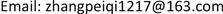1. 引言

2. 数据与指标2.1. 数据来源

2.2. 数据整理

U = [ u 1 p u 1 o u 1 h u 1 l u 1 c u 1 n u 1 v u 2 p u 2 o u 2 h u 2 l u 2 c u 2 n u 2 v u 3 p u 3 o u 3 h u 3 l u 3 c u 3 n u 3 v ⋮ ⋮ ⋮ ⋮ ⋮ ⋮ ⋮ u n p u n o u n h u n l u n c u n n u n v ] (1)

Δ c i = u i + m , c − u i , c , ∀ i = 1 , 2 , 3 , ⋯ , n − 1 (2)

y i = { − 1 ,     Δ c i < 0 1 ,         Δ c i ≥ 0 (3)

u i j = u i j − u min j u max j − u min j (4)

2.3. 技术分析指标

HSU  指出，在机器学习以前，金融经济学家一般更喜欢使用计量经济学，选择技术指标利用EMH模型来对股市进行分析。而技术指标依赖于过去的价格和成交量确定未来的价格趋势。现有的研究一般是基于多种技术指标构建交易策略提高盈利能力，包括过滤器规则  、移动平均线  、动量  和自动模式识别 。因此，本文在收集到的原始数据的基础上加入一些重要的技术指标构成最终的特征向量。加入的技术指标如表一所示：

U = [ u 1 p u 1 o u 1 h u 1 l u 1 c u 1 n u 1 v T 11 T 12 T 13 T 14 T 15 c 1 u 2 p u 2 o u 2 h u 2 l u 2 c u 2 n u 2 v T 21 T 22 T 23 T 24 T 25 c 2 u 3 p u 3 o u 3 h u 3 l u 3 c u 3 n u 3 v T 31 T 32 T 33 T 34 T 35 c 3 ⋮ ⋮ ⋮ ⋮ ⋮ ⋮ ⋮ ⋮ ⋮ ⋮ ⋮ ⋮ ⋮ u n p u n o u n h u n l u n c u n n u n v T n 1 T n 2 T n 3 T n 4 T n 5 c n ] (5)

2.4. 误差分析

MAPE = 1 N ∑ i = 1 N | u i , close − u ^ i , close u i , close | ∗ 100 (6)

RMSE = 1 N ∑ i = 1 N ( u i , close − u ^ i , close ) 2 (7)

3. 基于SVM-KNN的预测模型

3.1. 支持向量机分类算法

min Φ ( x ) = ‖ w ‖ 2 2 + C ∑ i = 1 n ξ i (7)

s.t { y i ( w T x i + b ) ≥ 1 − ξ i ξ i ≥ 0 ,   i = 1 , 2 , 3 , ⋯ , n

max Q ( α ) = ∑ i = 1 n α i − 1 2 ∑ i = 1 n ∑ j = 1 n α i α j y i y j 〈 ϕ ( x i ) , ϕ ( x j ) 〉 (8)

s.t { ∑ i = 1 n α i y i = 0 0 ≤ α i ≤ C , i = 1 , 2 , 3 , ⋯ , n

f ( x ) = sgn { ( w T ⋅ ϕ ( x ) ) } + b = sgn { ∑ 支 持 向 量 α i * y i 〈 ϕ ( x i ) , ϕ ( x ) 〉 + b * } (9)

K ( x i , x j ) = exp ( − γ ‖ x i − x j ‖ 2 ) , γ > 0 (10)

3.2. K近邻分类算法

KNN (K-Nearest-Neighbor)分类器是通过给定一个待识别的样本集，根据在训练集中寻找到最近的K个近邻，通过确定K的个数，从而将待识别样本进行归类。图2中给出的是在k = 5的条件下的分类状况。

3.3. SVM-KNN算法实现

Technical index calculation formul

4. 实例验证与结果分析

Parameter selection of SV

1天7天30天
C293652
γ0.20.70.7

SVM’s forecast of the ups and downs of the Shanghai Composite Index on different period

1天7天30天

MAPE and RMSE calculation error

1天7天30天

RMSE0.00510.00570.0102

5. 模拟投资

5.1. 构建投资策略

Thirty-day rise and fal

11/20112/4112/181
11/21112/5112/191
11/24112/8112/22−1
11/25112/9112/231
11/26−112/10112/24−1
11/27−112/11112/251
11/28−112/12112/26−1
12/1112/15112/29−1
12/2112/16112/30−1
12/3112/17112/31−1

5.2. 计算收益

t日实施投资并持有两天以上后将获得的股票当日收益率为：

R t = u t + n − u t u t (11)

CumR = ∏ t = 1 n ( 1 + R t ) − 1 (12)

5.3. 结果分析

Sharperatio = E ( R p ) − R f σ P (13)

6. 总结